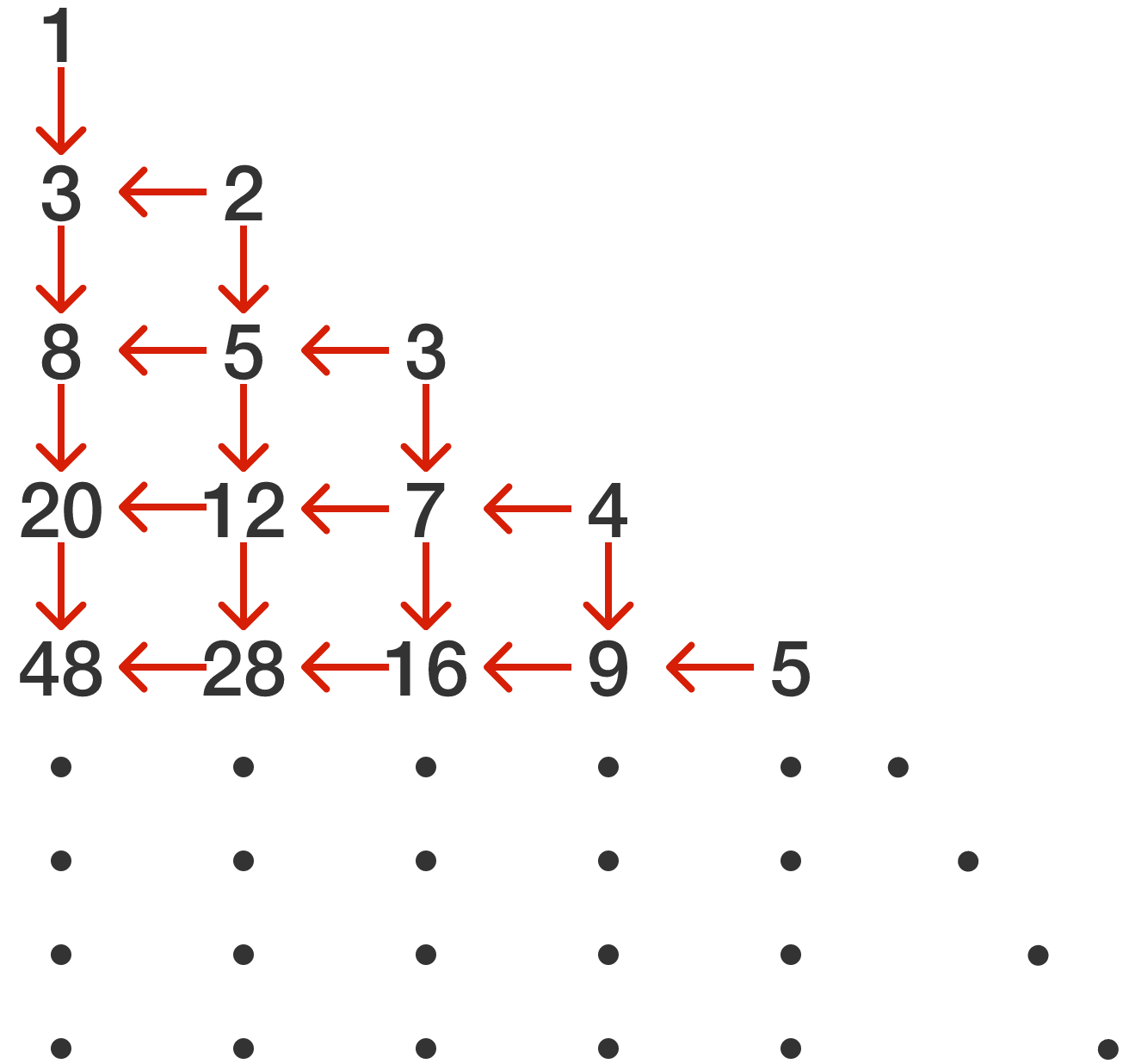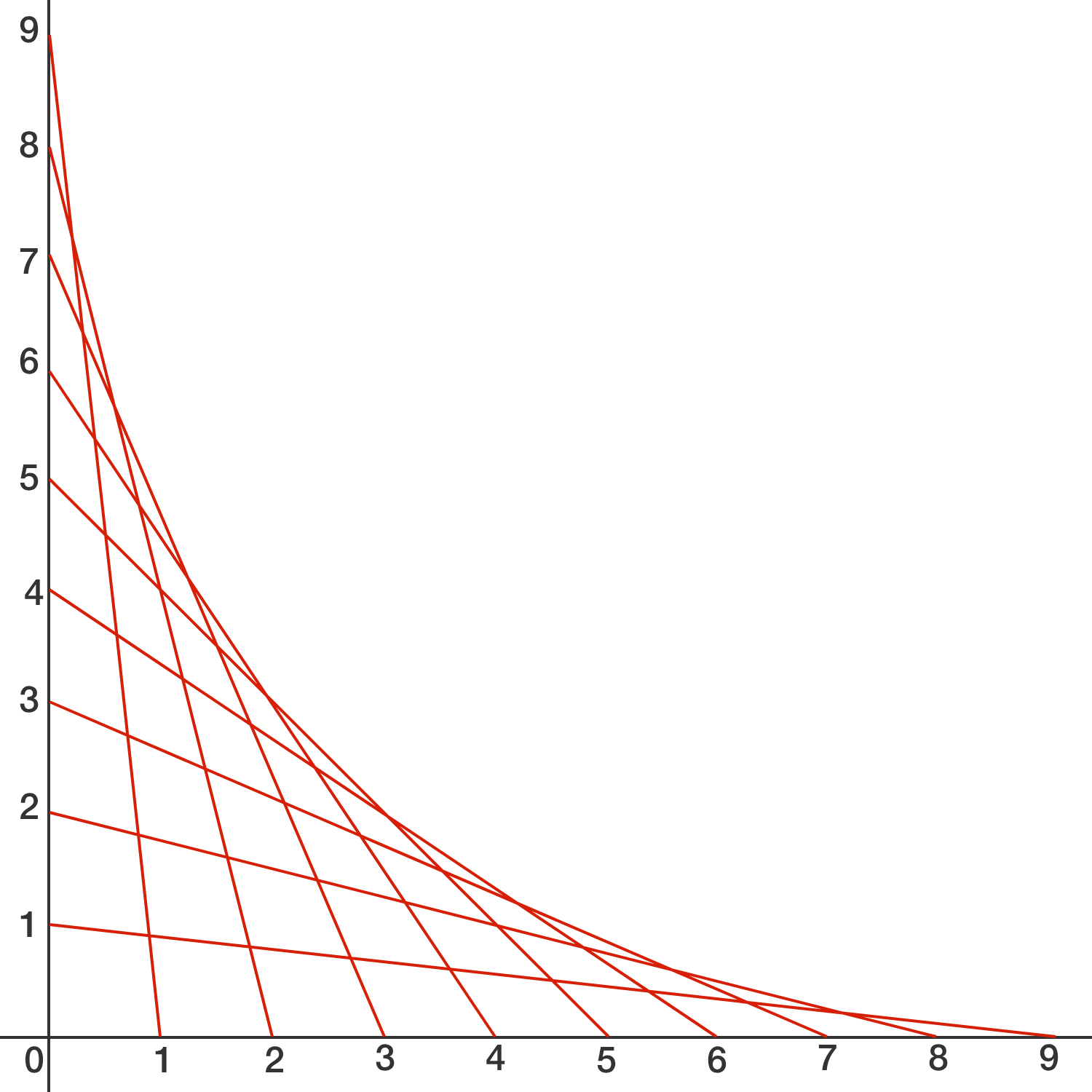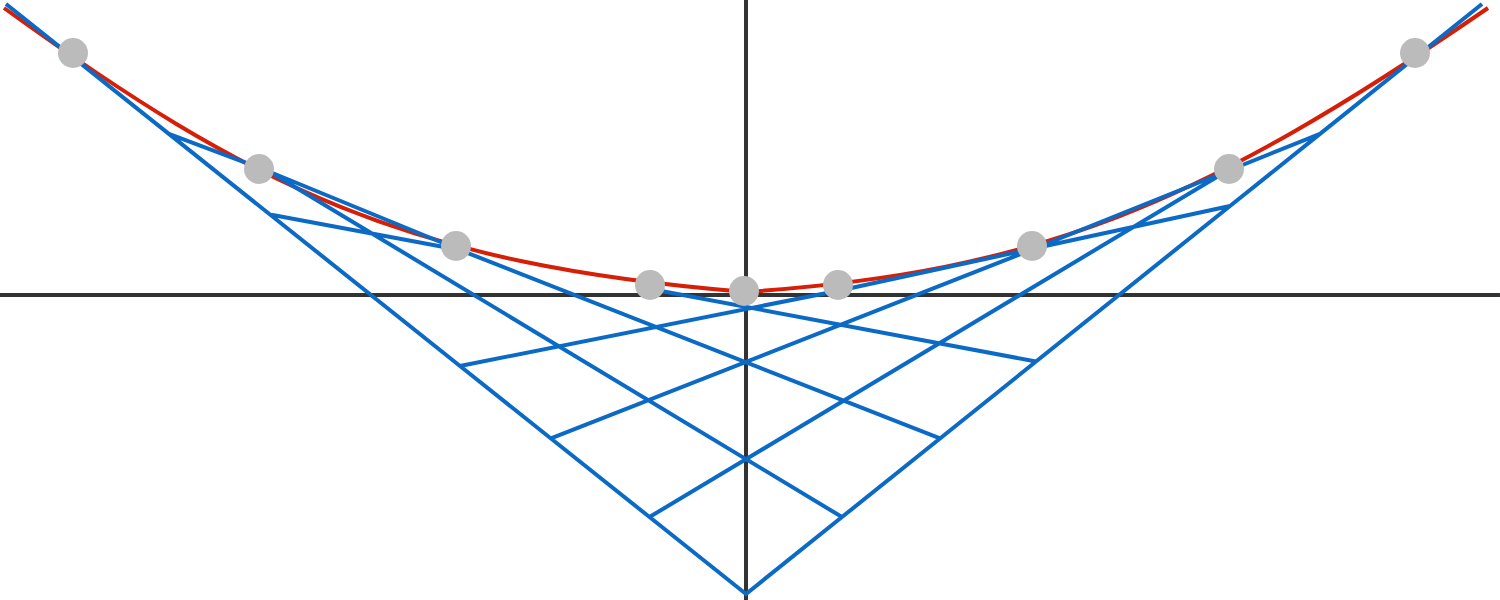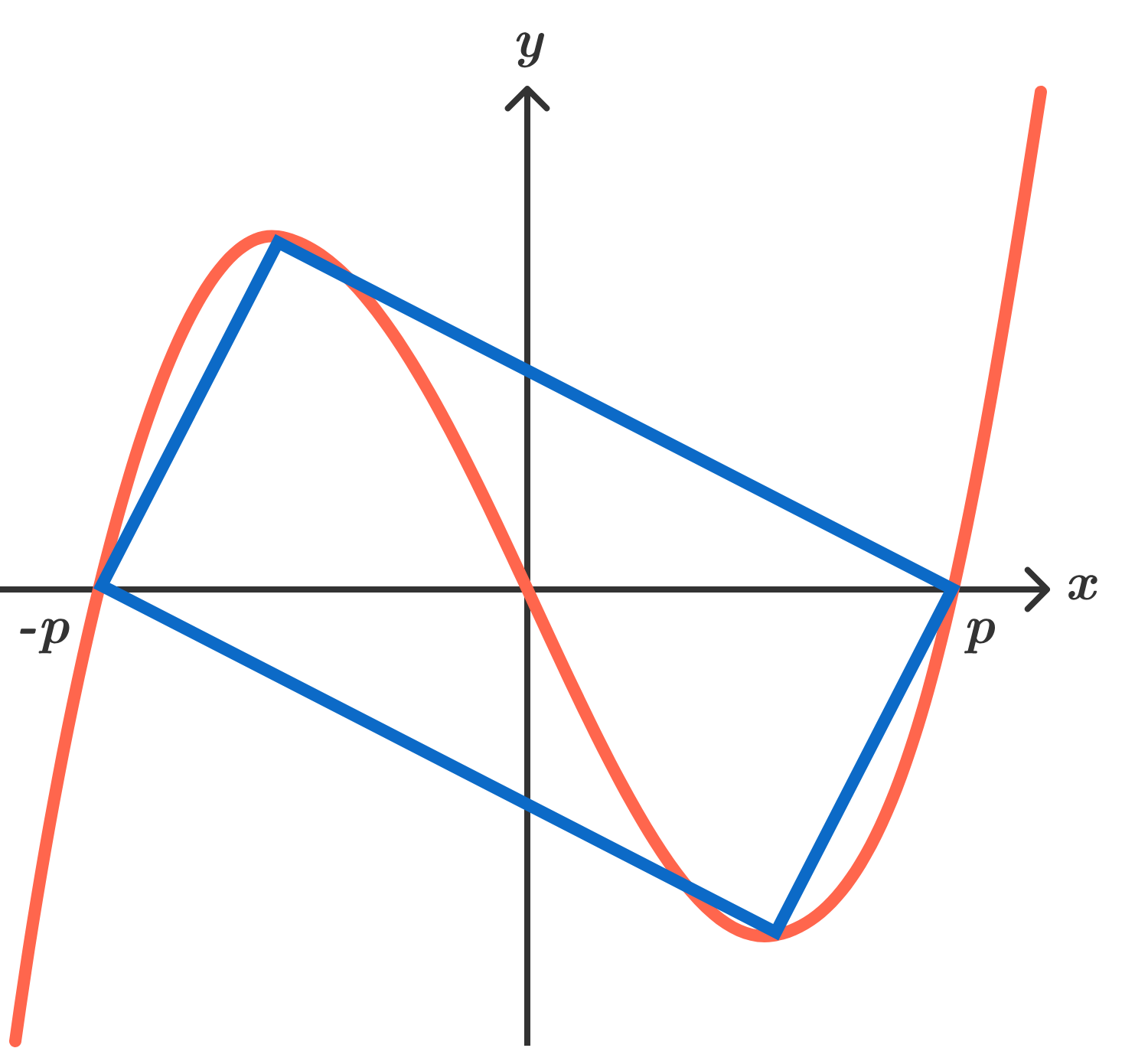# Problems of the Week

Contribute a problem

As we can see in involving involutions, there are a number of functions $f:\mathbb{R}\to\mathbb{R}$ that satisfy the relation

${\color{#69047E}f\big(f(x)\big)=}{\color{forestgreen}\, x} \ \ \forall x\in\mathbb{R}.$

Is there any function $f:\mathbb{R}\to\mathbb{R}$ with the property ${\color{#69047E}f\big(f(x)\big)=}{\color{#D61F06} -x} \ \ \forall x\in\mathbb{R}?$

We form a number triangle by first placing the positive integers along a down-and-right diagonal of an infinite square grid. Then, all other spaces in the triangle are filled by summing the numbers directly up and to the right of that space.The sequence $1,3,8,20,48,112,256,576,...$ occurs in the leftmost column of this triangle.

If $S(x)=x+3{ x }^{ 2 }+{ 8 }x^{ 3 }+{ 20x }^{ 4 }+48{ x }^{ 5 }+112{ x }^{ 6 }+256{ x }^{ 7 }+576{ x }^{ 8 }+\cdots,$ what is $S\big(\frac13\big)?$

Bonus: Generalize $S(n).$The above curve-stitching is formed by joining $(i,0)$ to $(0,10-i)$ for $i = 1, 2, \ldots, 9$.

If $i$ is all the numbers between 1 and 10, not just integers, the outer curve will be smooth rather than kinked.

Now, after a rotation and a translation shown below, this smooth curve can be expressed as $y = \frac{\sqrt{a}}{{b}}x^2$, where $a$ and $b$ are positive integers with $a$ square-free.

What is the value of $\frac{b^2}{a}?$If a cubic equation has real zeroes $\pm p$ and 0, and its two horizontal tangent points and two non-zero $x$-intercepts can be joined together to form a rectangle (as pictured), then the ratio of the rectangle’s larger side to its shorter side can be expressed as $\frac{\sqrt{a} + \sqrt{b}}{c}$, where $a$, $b$, and $c$ are square-free integers.

What is $a + b + c?$

• $\sqrt{a} + \sqrt{b}$ is a root of $10x^3 - 30x - 29$.
• $\sqrt{a} + \sqrt{b}$ is a root of $10x^5 - 50x^3 + 50x - 29$.
• $\sqrt{a} + \sqrt{b}$ is a root of $n_1x^7 + n_2x^5 + n_3x^3 + n_4x + n_5$.

If $\gcd(n_1, n_2, n_3, n_4, n_5) = 1$ with $n_1 > 0$, what is the value of $|n_1|+|n_2|+|n_3|+|n_4|+|n_5|?$

×